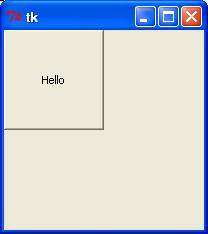# Python 3 - Tkinter place() Method

This geometry manager organizes widgets by placing them in a specific position in the parent widget.

## Syntax

```widget.place( place_options )
```

Here is the list of possible options −

• anchor − The exact spot of widget other options refer to: may be N, E, S, W, NE, NW, SE, or SW, compass directions indicating the corners and sides of widget; default is NW (the upper left corner of widget)

• bordermode − INSIDE (the default) to indicate that other options refer to the parent's inside (ignoring the parent's border); OUTSIDE otherwise.

• height, width − Height and width in pixels.

• relheight, relwidth − Height and width as a float between 0.0 and 1.0, as a fraction of the height and width of the parent widget.

• relx, rely − Horizontal and vertical offset as a float between 0.0 and 1.0, as a fraction of the height and width of the parent widget.

• x, y − Horizontal and vertical offset in pixels.

## Example

Try the following example by moving cursor on different buttons −

```# !/usr/bin/python3
from tkinter import *

top = Tk()
L1 = Label(top, text = "Physics")
L1.place(x = 10,y = 10)
E1 = Entry(top, bd = 5)
E1.place(x = 60,y = 10)
L2 = Label(top,text = "Maths")
L2.place(x = 10,y = 50)
E2 = Entry(top,bd = 5)
E2.place(x = 60,y = 50)

L3 = Label(top,text = "Total")
L3.place(x = 10,y = 150)
E3 = Entry(top,bd = 5)
E3.place(x = 60,y = 150)

B = Button(top, text = "Add")
B.place(x = 100, y = 100)
top.geometry("250x250+10+10")
top.mainloop()
```

When the above code is executed, it produces the following result −python_gui_programming.htm